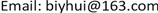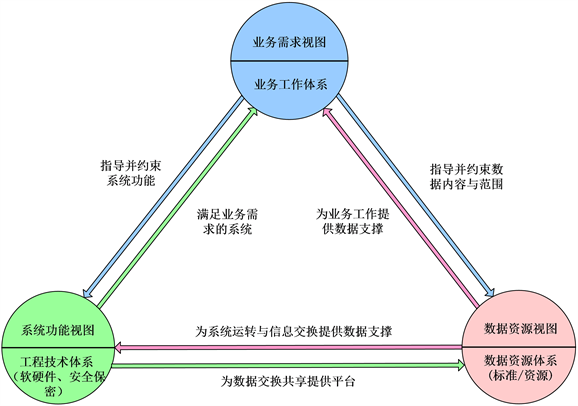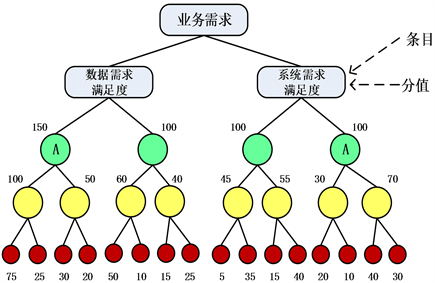﻿ 一种基于三视图模型的数据中心建设质量评估方法研究 Study on an Evaluation Method of Data Center Construction Quality Based on Three-View Model

Computer Science and Application
Vol. 09  No. 10 ( 2019 ), Article ID: 32614 , 6 pages
10.12677/CSA.2019.910213

Study on an Evaluation Method of Data Center Construction Quality Based on Three-View Model

Zhong Tian, Yuhui Bi

The Army of 31008, BeijingReceived: Oct. 1st, 2019; accepted: Oct. 16th, 2019; published: Oct. 23rd, 2019ABSTRACT

A three-view evaluation indicator model based on requirement, system and data was proposed in the paper according to the feature of data center construction, and then evaluation indicators system and indicator weights were designed, and judged through fuzzy comprehensive evaluation model. The method can provide some reference for the evaluation of the quality of data center construction.

Keywords:Data Center Construction, Evaluation Indicators, Quality Evaluation

31008部队，北京1. 引言

2. 评估指标体系构建原则

3. 三视图指标体系模型Figure 1. Model: requirement, system and data

4. 评估指标构建

5. 指标权重方法确定Figure 2. Indicators weight design

6. 评估计算方法设计

$R=\left(\begin{array}{c}{B}_{1}\\ {B}_{2}\\ ⋮\\ {B}_{i}\end{array}\right)=\left(\begin{array}{c}{b}_{11}\\ {b}_{21}\\ \cdots \\ {b}_{i1}\end{array}\begin{array}{c}{b}_{12}\\ {b}_{22}\\ \cdots \\ {b}_{i2}\end{array}\begin{array}{c}{b}_{13}\\ {b}_{23}\\ \cdots \\ {b}_{i3}\end{array}\begin{array}{c}{b}_{14}\\ {b}_{24}\\ \cdots \\ {b}_{i4}\end{array}\right)$ (1)

$X={Q}^{\prime }×{V}^{\prime }$ (2)

$X=\left(0.29,0.35,0.21,0.15\right)\left(\begin{array}{c}100\\ 80\\ 60\\ 40\end{array}\right)=75.6$

7. 结语

Study on an Evaluation Method of Data Center Construction Quality Based on Three-View Model[J]. 计算机科学与应用, 2019, 09(10): 1906-1911. https://doi.org/10.12677/CSA.2019.910213

1. 1. 董红磊, 陈玉忠, 张金换. 产品缺陷风险评价指标体系和方法研究[J]. 标准科学, 2016(5): 80-83.

2. 2. 张凌, 李巧玲, 文锦军, 杨新章. 面向用户的数据中心发展模式探讨[J]. 广东通信技术, 2018, 38(11): 28-31.

3. 3. 国防科学技术大学信息系统与管理学院. 体系结构研究[M]. 北京: 军事科学出版社, 2011: 56-80.

4. 4. DOD Architec-ture Framework Working Group (2009) DOD Architecture Framework Version 2.0 Volume 1: Introduction, Overview, and Concepts. Department of Defense, US.

5. 5. DOD Architecture Framework Working Group (2009) DOD Archi-tecture Framework Version 2.0 Volume 2: Architecture Data and Models. Department of Defense, US.

6. 6. 连玉明. 基于新发展理念的高质量发展“1 + 5”评价指标体系[J]. 大数据新时代, 2019(2): 20-61.

7. 7. 杜东, 易文安, 高博. 基于大数据的军校学员综合素质评估模型构建研究[C]//第二届军事大数据论坛, 2019: 399-403.

8. 8. 杜金环, 金璐璐. 软件质量度量过程及模型研究[J]. 计算机技术与发展, 2014, 24(4): 38-40.

9. 9. 罗玫, 赵嵩正, 蒋建洪. 模糊综合评价模型的R语言实现[J]. 航空计算技术, 2011, 41(4): 26-29.

10. 10. 顾宇. 基于层次分析法和模糊综合评价法的项目管理成熟度模型应用[J]. 科技创新与应用, 2018, 254(34): 172-173.